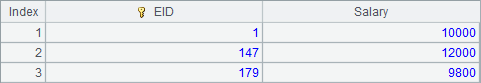• 集算器
教程
函数参考
习题
代码参考
用户参考
外部库使用指南
数据文件工具用户参考
DQL教程
集群管理器使用说明
SPL命令执行器用户参考
• 润乾报表
教程
填报教程
分析教程
报表中心教程
用户参考
程序员参考
高级指南
通用查询
DQL部署集成手册
• 易明建模
用户参考
json参数说明
• 润乾官网

# 虚表

## 虚表的基本使用

 A B 1 =demo.query("select NAME, SURNAME, GENDER, STATE from employee") 2 =A1.select(GENDER=="M") =A1\A2 3 =A2.(NAME).id() =A3.len() 4 =B2.(NAME).id() =A4.len() 5 =A1.(SURNAME).id() =A5.len() 6 =A1.(STATE).id() =A6.len() 7 [Sales,Technology,R&D,Financial,Admin] [0,0.5,0.75,0.9,0.97,1] 8 =to(1000).new(#:EID, B7.pseg(rand()):DeptID, if(rand()<0.5,0,1):Gender, if(rand()>0.8,1,0):Married, if(Gender==0,A3(rand(B3)+1), A4(rand(B4)+1))/" "/A5(rand(B5)+1):Name, bits(Gender, Married):Bools ) 9 =A8.select(DeptID==1) =A9.len() 10 2020-01-01 2021-01-01 11 =periods@x(A10,B10) =periods@x(B10,elapse@y(B10,1)) 12 =A11.((a=string(~,"yyMMdd"), to(rand(100)+ 10).new(A9(if(rand()>0.9, rand(B9)+1, rand(B9-20)+ 21)).EID:SID, a/string(#, "0000"):OID, datetime(A11.~, time(rand(8)+8, rand(60), 0)):OTime, rand(100)*10+200:Amount))).conj().sort(SID, OID) 13 =B11.((a=string(~,"yyMMdd"), to(rand(100)+ 10).new(A9(if(rand()>0.9, rand(B9)+1, rand(B9-20)+ 21)).EID:SID, a/string(#, "0000"):OID, datetime(B11.~, time(rand(8)+8, rand(60), 0)):OTime, rand(100)*10+200:Amount))).conj().sort(SID, OID) 14 =file("D:/file/pseudo/emps.ctx") =A14.create(#EID,DeptID,Gender, Married, Name, Bools) 15 >B14.append@i(A8.cursor()) 16 =file("D:/file/pseudo/1.orders.ctx") =file("D:/file/pseudo/2.orders.ctx") 17 =A16.create(SID, #OID, OTime, Amount) >A17.append(A12.cursor()) 18 =B16.create(SID, #OID, OTime, Amount) >A18.append(A13.cursor()) 19 >B14.close() 20 >A17.close() >A18.close()A12A13中构建的20202021年度的订单表数据如下：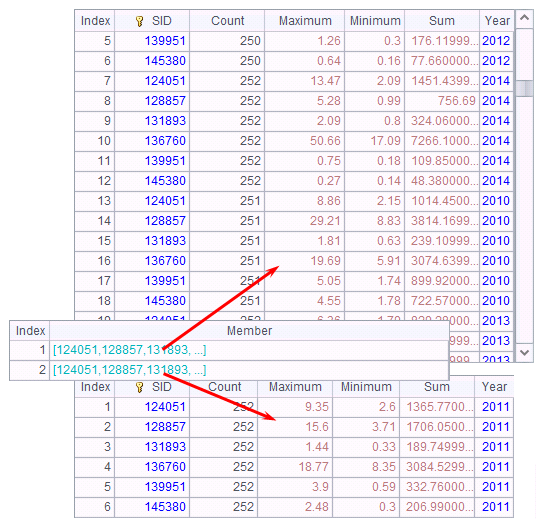A B C 1 =create(file).record(["pseudo/emps.ctx"]) =pseudo(A1) =B1.cursor().fetch@x(100) 2 =create(file, zone).record(["pseudo/orders.ctx", [1,2]]) =pseudo(A2) =B2.import()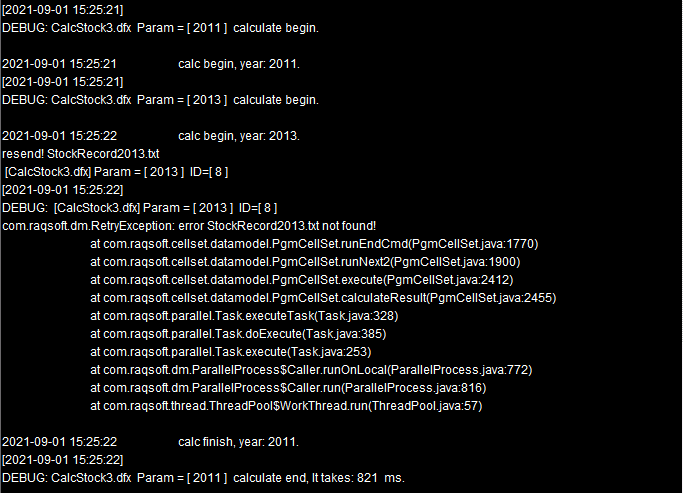C2从虚表中获取到的数据如下：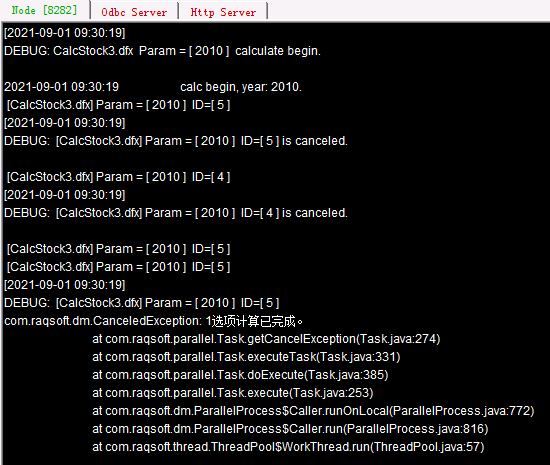A B 1 =create(file).record(["pseudo/emps.ctx"]) =pseudo(A1) 2 =B1.select(DeptID==2 && EID<10) =A2.import() 3 >B2.run(DeptID=0) =B1.update(B2) 4 =B1.import() =A2.import() 5 >B1.delete(B2) =B1.import() 6 >B2.run(DeptID=2, EID=EID+1000) =B1.append(B2.cursor()) 7 =B1.import()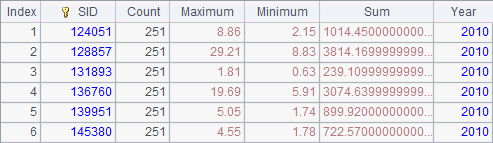A3中把这些员工记录的DeptID字段改为0，并在B3中用T.update(P)函数将修改后的排列P中的记录更新到虚表中。使用delete/update/append等函数对虚表进行维护操作时，虚表对应的组表必须定义维，对虚表的维护操作都是针对组表的维执行的。更新后，A4B4中得到的数据如下：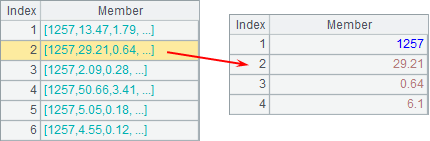A5中用T.delete(P)函数，在虚表T中将排列P中的记录删除，删除时也是根据组表的维执行的，执行后，在B5中从虚表中获取数据如下：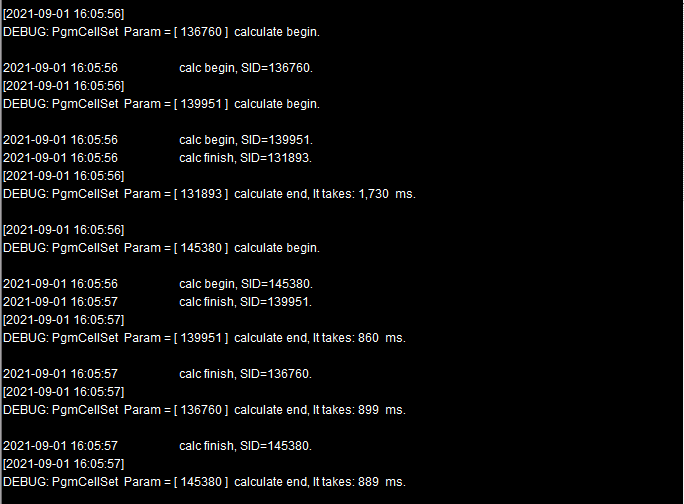A6中将B2中结果中两位员工的DeptID改回原值，同时把EID增加1000，并用T.append(cs)函数用虚表构建游标添加到虚表中，A7中结果如下：A B 1 =create(file).record(["D:/files/txt/PersonnelInfo.btx "]) =pseudo(A1) 2 =B1.cursor().fetch@x(100) 3 =B1.select(City=="Columbus" && ID<500) =A3.import()

A2中用游标访问虚表，结果如下：A3中从虚表中选择出Columbu市且编号在500以内的员工，B3结果如下：## 虚表的归并

 A 1 =create(file,zone).record(["pseudo/orders.ctx", [1,2]]) 2 =pseudo(A1) 3 =A2.import() 4 =A2.group(year(OTime)).import() 5 =A4.new(SID,year(OTime):Year,sum(Amount):Total) 6 =A2.groups(SID, year(OTime):Year;sum(Amount):Total)

A1中的虚表定义记录如下：A4中按照订单年份执行分组，结果如下：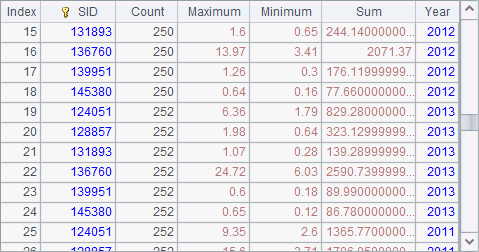A 1 =create(file,zone,user,date).record(["pseudo/orders.ctx", [1,2],"SID","OTime"]) 2 =pseudo(A1) 3 =A2.import() 4 =A2.group(year(OTime)).import() 5 =A4.new(SID,year(OTime):Year,sum(Amount):Total) 6 =A2.select(OTime>date(2020,12,20) && OTime

A1中虚表定义记录如下：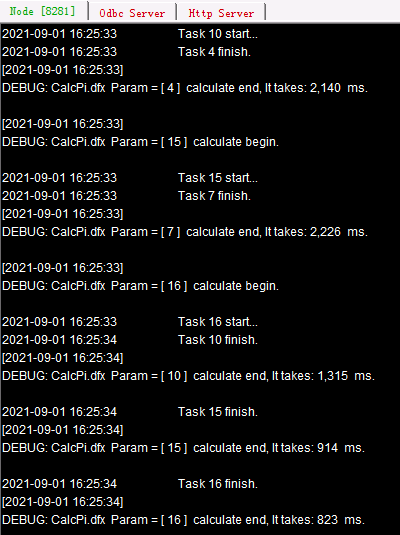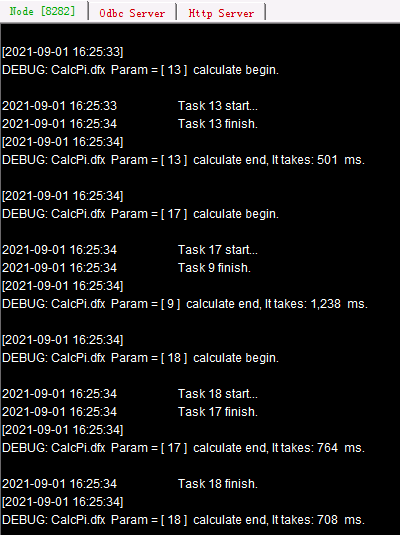A4中按照订单年份执行分组，结果如下：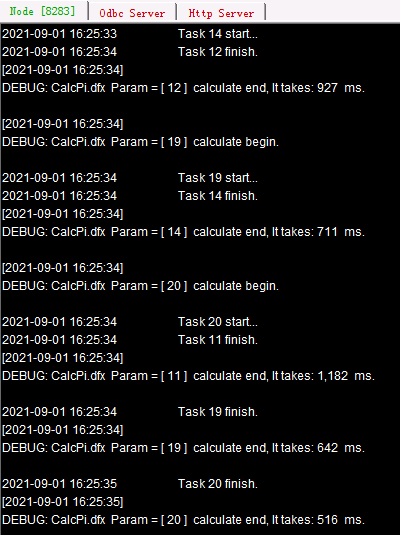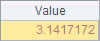A6中，查询日期在20201220日起至2021110日前的订单，A7中获取数据如下：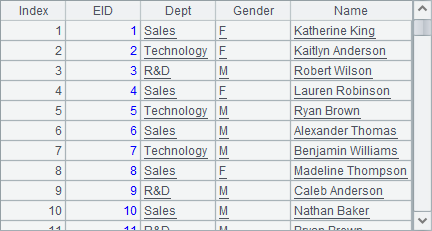A B 1 =demo.query("select distinct(NAME) from CITIES") =A1.(NAME) 2 =create(file,zone).record(["pseudo/orders.ctx", ]) =pseudo(A2).import() 3 =create(file,zone).record(["pseudo/orders.ctx", ]) =pseudo(A3).import() 4 =B1.len() =1000.(B1(rand(A4)+1)) 5 =B2.new(B4(SID):City, SID, OID, OTime, Amount) =B3.new(B4(SID):City, SID, OID, OTime, Amount) 6 =A5.sort(City) =B5.sort(City) 7 =file("pseudo/1.orders2.ctx") =file("pseudo/2.orders2.ctx") 8 =A7.create(City, SID, #OID, OTime, Amount) >A8.append(A6.cursor()) 9 =B7.create(City, SID, #OID, OTime, Amount) >A9.append(B6.cursor())

 A B 1 =create(file,zone, date).record(["pseudo/orders2.ctx", [1,2], "OTime"]) =pseudo(A1).import() 2 =create(file,zone, user, date).record(["pseudo/orders2.ctx",  [1,2] , "SID", "OTime"]) =pseudo(A2).import()

A1中的虚表从复组表中取数，数据将按首字段归并，B1中结果如下：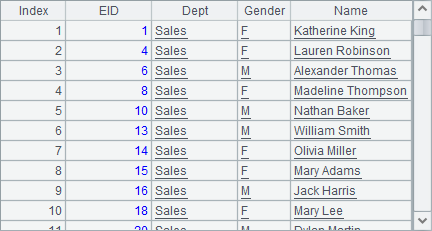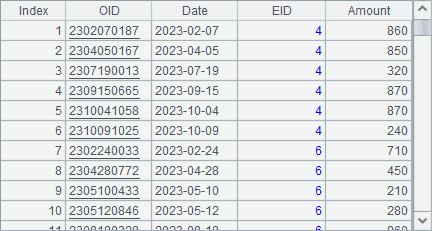## 用户定义字段

 A 1 [Sales,Technology,R&D,Financial,Admin] 2 =create(name,pseudo,enum).record(["DeptID","Dept",A1]) 3 =create(name,bits).record(["Bools",["IfMarried","IfLady"]]) 4 =create(file,column).record(["pseudo/emps.ctx",A2|A3]) 5 =pseudo(A4) 6 =A5.select(Dept=="Sales").import() 7 =A5.import(EID, Name, DeptID, Dept) 8 =A5.select(!IfLady && IfMarried).import() 9 =A5.import(EID, Name, Gender, IfLady,Married,IfMarried)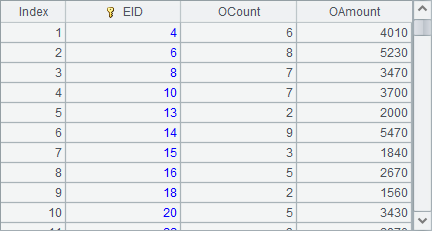column定义记录中，name字段是字段的名称，如果是原始数据表中的字段，称为真字段，除此之外还可能是其它列生成的伪字段。如果需要把真字段DeptID转换为对应的部门名称，这样的伪字段是枚举伪字段，在column记录中用pseudo字段定义枚举伪字段的名称，部门转换时需要把对应的需要转换为序列中的值，转换时对应的取值序列需要在enum列中设置，转换时将把对应的编号转换为对应的值，位置1开始

A3中定义了另一个用户定义字段，原始数据表中，Bools字段存储了2个二值型字段的数据，性别和是否已婚。A3中字段定义记录如下：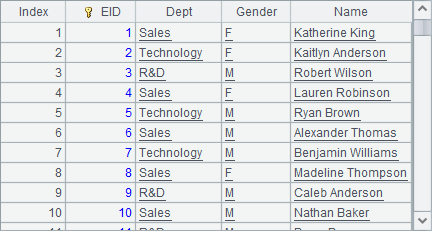A4中定义虚表，设置了数据文件file和字段定义column，如下：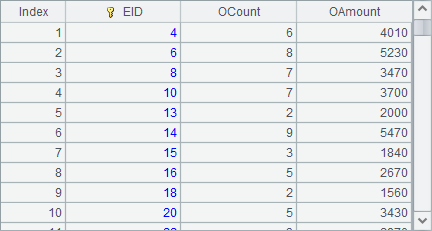A5中使用这个定义记录生成虚表，在这个虚表中，就可以使用定义的column了。如A6中使用枚举伪字段Dept来筛选出销售部员工如下：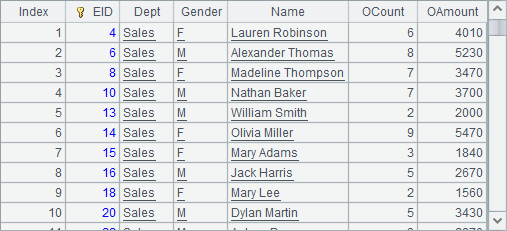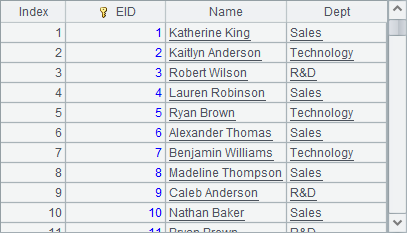A8中使用二值维度伪字段执行筛选，选出已婚男员工的数据，结果如下：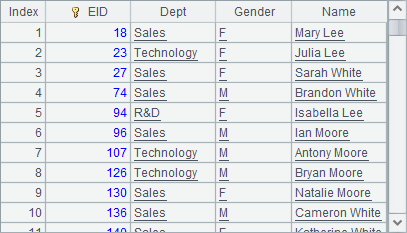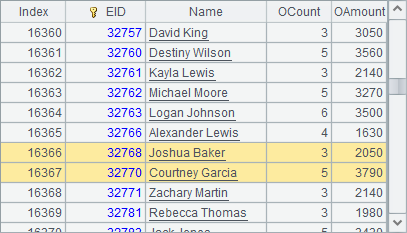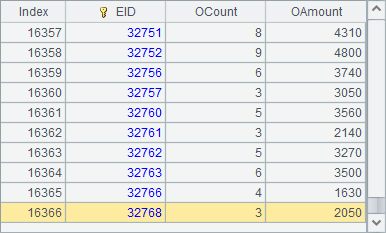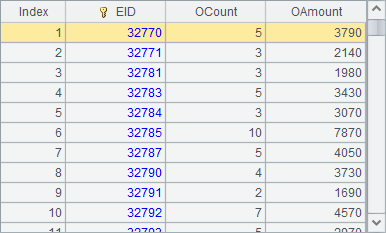A11中，可以看到虚表使用的组表文件中，数据实际被修改的情况：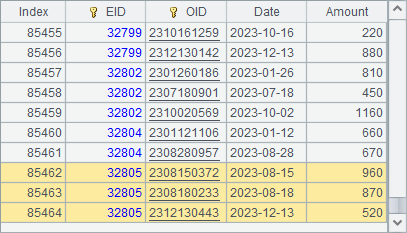A 1 =create(file).record(["pseudo/emps.ctx"]) 2 =pseudo(A1).select(DeptID==1).memory().keys(EID) 3 =create(name,dim,fkey).record(["SID",A2,["SID"]]) 4 =create(name,exp).record(["OYear","year(OTime)"]) 5 =create(file,zone,column).record(["pseudo/orders.ctx",,A3|A4]) 6 =pseudo(A5) 7 =A6.select(left(SID.Name, 4)=="Jack").import(SID, OTime, OYear, Amount) 8 =A7.new(SID.EID:SID, SID.Name:Name, OTime, OYear, Amount) 9 =A6.import(SID.EID:SID, SID.Name:Name, OTime, OYear, Amount)

A2中从员工虚表中获取销售部的人员数据，并用T.memory()将其加载为内表，并设主键为员工编号EID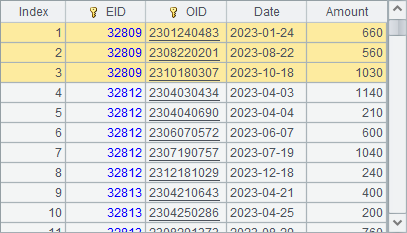A3中添加用户定义字段：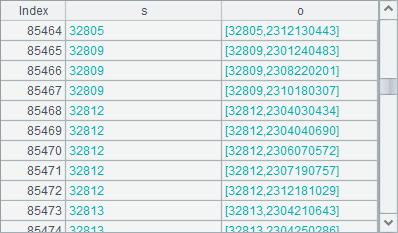A4中添加用户定义字段：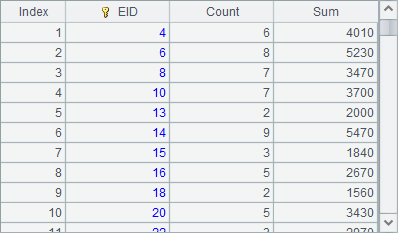A5中的虚表定义记录如下：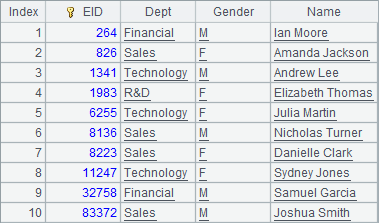A7中从虚表中，获取名为Jack的员工的销售记录如下：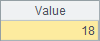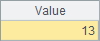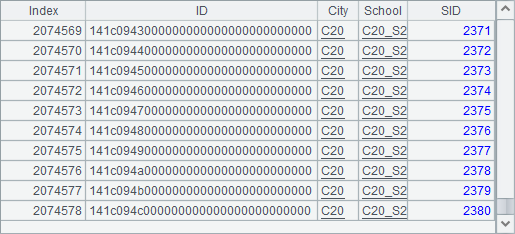A B 1 =demo.query("select distinct(NAME) from CITIES") =A1.(NAME) 2 =create(City, FactoryID, PTime) =8.(elapse@m(date(2020,1,1), (~-1)*3)+rand(3)) 3 >B1.run(B2.(if(#<2 || rand()>0.5, A2.insert(0, B1.~, rand(5)+1, B2.~)))) >file("pseudo/FacSupply.btx").export@b(A2) 4 =create(name,dim,fkey,tkey).record(["Product",A2, ["City"], "OTime"]) >A2.keys@t(City;PTime) 5 =create(file,zone,user,date,column).record(["pseudo/orders2.ctx",[1,2],"SID","OTime", A4]) =pseudo(A5) 6 =A5.select(Amount>1100) =A6.import() 7 =A6. import(City, SID, OTime, Amount, Product.FactoryID:Factory, Product.PTime:PTime)

B1中生成各个城市名构成的序列，A2中新建产品供应表，在A3中准备测试数据，由5个工厂随机供货，在每季度初可能向某个城市供货，而202011日所有城市都需要完成初始供货。A3中数据填入后，A2中供货记录如下：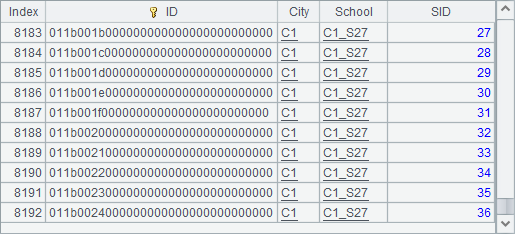A4中添加用户定义字段：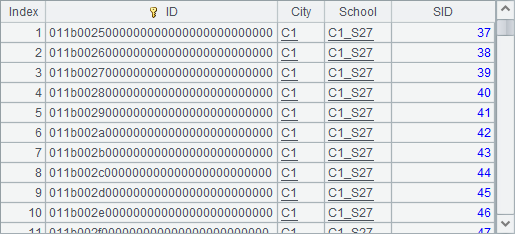A5中的虚表定义记录如下：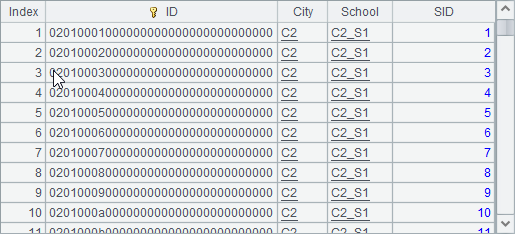B5中使用这个虚表定义建立虚表后，在A6中从中查询出Amount大于1100的销售记录，B6取出数据如下：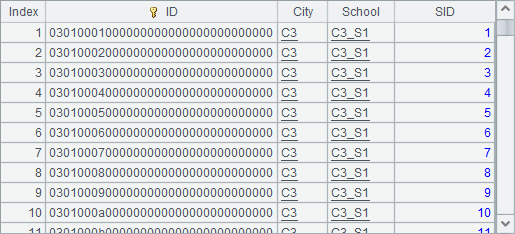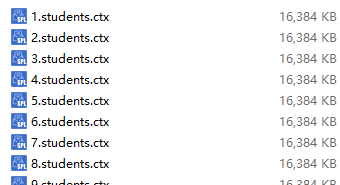A 1 >tab=demo.query("select * from CITIES") 2 =create(var).record(["tab"]) 3 =pseudo(A2) 4 =A3.select(STATEID==3) 5 =A4.import()

A1中从数据库中获取CITIES表并将序表存储到变量tabA2使用内存表来定义虚表。A4中从虚表中获取STATEID3的城市资料，A5中得到的结果如下：